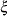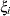Checking Convergence in FCS Methods

The parameters used in the imputation step at each iteration can be saved in an output data set with the OUTITER= option. These include the means and standard deviations. You can then monitor the convergence by displaying trace plots for those parameter values with the PLOTS=TRACE option.

A trace plot for a parameteris a scatter plot of successive parameter estimatesagainst the iteration number. The plot provides a simple way to examine the convergence behavior of the estimation algorithm for. Long-term trends in the plot indicate that successive iterations are highly correlated and that the series of iterations has not converged.

You can display trace plots for the variable means and standard deviations. You can also request logarithmic transformations for positive parameters in the plots with the LOG option. With the LOG option, if a parameter value is less than or equal to zero, then the value is not displayed in the corresponding plot.

See Example 56.8 for a usage of the trace plot.